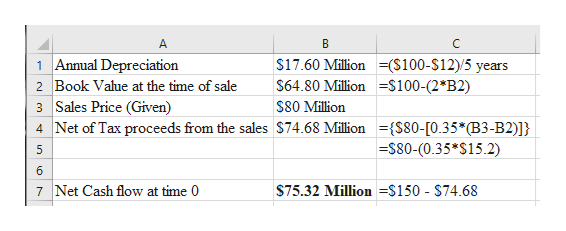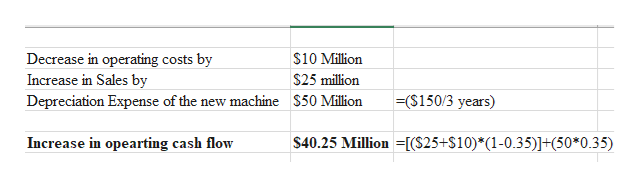PC Shopping Network may upgrade its modem pool. It last upgraded 2 years ago, when it spent \$100 million on equipment with an assumed life of 5 years and an assumed salvage value of \$12 million for tax purposes. The firm uses straight-line depreciation. The old equipment can be sold today for \$80 million. A new modem pool can be installed today for \$150 million. This will have a 3-year life and will be depreciated to zero using straight-line depreciation. The new equipment will enable the firm to increase sales by \$25 million per year and decrease operating costs by \$10 million per year. At the end of 0 years, the new equipment will be worthless. Assume the firm’s tax rate is 35% and the discount rate for projects of this sort is 10%. a. What is the net cash flow at time 0 if the old equipment is replaced? (Negative amounts should be indicated by a minus sign. Do not round intermediate calculations. Enter your answer in millions rounded to 2 decimal places.) b.What are the incremental cash flows in years 1, 2, and 3? (Do not round intermediate calculations. Enter your answer in millions rounded to 2 decimal places.) c.What are the NPV and IRR of the replacement project? (Do not round intermediate calculations. Enter the NPV in millions rounded to 2 decimal places. Enter the IRR as a percent rounded to 2 decimal places.)

Question

PC Shopping Network may upgrade its modem pool. It last upgraded 2 years ago, when it spent \$100 million on equipment with an assumed life of 5 years and an assumed salvage value of \$12 million for tax purposes. The firm uses straight-line depreciation. The old equipment can be sold today for \$80 million. A new modem pool can be installed today for \$150 million. This will have a 3-year life and will be depreciated to zero using straight-line depreciation. The new equipment will enable the firm to increase sales by \$25 million per year and decrease operating costs by \$10 million per year. At the end of 0 years, the new equipment will be worthless. Assume the firm’s tax rate is 35% and the discount rate for projects of this sort is 10%.

a. What is the net cash flow at time 0 if the old equipment is replaced? (Negative amounts should be indicated by a minus sign. Do not round intermediate calculations. Enter your answer in millions rounded to 2 decimal places.)

b.What are the incremental cash flows in years 1, 2, and 3? (Do not round intermediate calculations. Enter your answer in millions rounded to 2 decimal places.)

c.What are the NPV and IRR of the replacement project? (Do not round intermediate calculations. Enter the NPV in millions rounded to 2 decimal places. Enter the IRR as a percent rounded to 2 decimal places.)

Step 1

Net cash flow: The difference between the company’s inflow and outflow of cash calculated for a given period is called as net cash flow.

NPV: Net Present Value is the difference between the present value of inflows and outflows of cash, which is recorded for a given period of time. It is a helpful measure for analyzing the profitability of the project which requires investment.

IRR: Internal Rate of Return is also used to measure the profitability of the invested project. It is a discounting rate, and eventually. It makes the NPV of the project’s cash flows equal to 0 (zero).

Step 2

The depreciation method used by the firm is SLM (Straight Line Method).

a. Calculation of Net Cash Flow at the time 0, if the old equipment is replaced is shown below:

Hence, the cash flow (outflow) at time 0, when the old equipment is replaced is (\$75.32) Million.

Note: By the help of annual depreciation calculated, the book value of the equipment at the time of sale will be calculated. Further, the net tax proceeds will be calculated at the given tax rate of 35%.help_outlineImage TranscriptioncloseA B C \$17.60 Million(\$100-\$12)5 years \$64.80 Million -S100-(2*B2) 1 Annual Depreciation Book Value at the time of sale 2 3 Sales Price (Given) 4 Net of Tax proceeds from the sales \$74.68 Million ={S80-[0.35*(B3-B2)]} \$80 Million \$80-(0.35*S15.2) 5 6 7 Net Cash flow at time 0 \$75.32 Million =\$150 - \$74.68 LO LO fullscreen
Step 3

b. Calculation of incremental cash flows for the year 1,2 and 3 is shown below:

Hence, the amount of ...help_outlineImage TranscriptioncloseDecrease in operating costs by Increase in Sales by \$10 Million \$25 million (\$150/3 years) Depreciation Expense of the new machine \$50 Million \$40.25 Million =[(\$25+S10)*(1-0.35)]+(50*0.35) Increase in opearting cash flow fullscreen

Want to see the full answer?

See Solution

Want to see this answer and more?

Our solutions are written by experts, many with advanced degrees, and available 24/7

See Solution
Tagged in